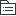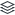刑法

|
10

相关模板推荐

•刑法 —— 作品大纲

•概述
• 概念
• 基本原则（三条）
• 犯罪的概念与特征
•犯罪的构成要件
• 犯罪主体
• 自然人犯罪
• 自然人的刑事责任年龄（14、16）
• 自然人的刑事责任能力（无，限制，完全）
• 单位犯罪
• 特征（3），处罚原则（双罚，单罚）
• 不以单位犯罪论处（3）
• 犯罪主观方面
• 犯罪故意
• 直接故意
• 间接故意
• 犯罪过失
• 疏忽大意
• 过于自信
• 不可抗力与意外事件
• 犯罪客体（犯罪构成的必要要件）
• 犯罪客观方面
• 犯罪行为
• 作为
• 不作为（四个特点义务来源）
• 犯罪结果
• 因果关系
•犯罪排除事由
• 正当防卫
• 概念
• 构成条件（5条）
• 防卫过当及其处罚
• 特殊正当防卫（无限防卫权）
• 紧急避险
• 概念
• 构成要件（避险对象）
•犯罪故意的形态
• 犯罪完成的形态
• 犯罪既遂
• 犯罪未完成的形态
• 犯罪预备
• 概念
• 特征
• 处罚原则
• 犯罪未遂
• 概念
• 特征
• 处罚原则
• 犯罪中止
• 概念
• 特征
• 处罚原则
•共同犯罪（两人以上故意）
• 主犯
• 从犯
• 胁从犯
• 教唆犯
•刑罚
• 主刑
• 管制
• 概念和特征
• 期限（3个月-2年，<=3年）
• 拘役
• 1-6个月
• 两项待遇
• 有期徒刑
• 6个月-15年
• 无期徒刑
• 死刑
• 适用对象例外
• 核准和执行
• 死缓
• 含义
• 期满处理（累犯，八大罪限制减刑）
• 附加刑
• 罚金
• 剥夺政治权利
• 没收财产
• 驱逐出境
• 累犯
• 一般累犯
• 特殊累犯
• 缓刑
• 适用条件
• 考验期
•刑法分论（常见罪名）
• 交通肇事罪与危险驾驶罪
• 交通肇事罪
• 危险驾驶罪
• 侵犯知识产权罪
• 侵犯著作权罪
• 侵犯商业秘密罪
• 侵犯公民人身权利、民主权利罪
• 故意杀人最与过失致人死亡罪（心态区别）
• 故意伤害罪与过失致人重伤罪
• 强奸罪
• 绑架罪（撕票无期或死刑）
• 侵犯财产罪
• 抢劫罪
• 八个情形加重处罚，可死刑
• 转化型抢劫罪（盗窃，诈骗，抢夺）
• 抢夺罪
• 盗窃罪（失控加控制说）
• 诈骗罪
• 侵占罪与职务侵占罪
• 贪污受贿罪
• 贪污罪
• 概念（主题特殊）
• 处罚
• 30-100万 三年十年
• 挪用公款罪
• 受贿罪与利用影响力受贿罪
• 受贿罪
• 利用影响力受贿罪
• 行贿罪与对有影响力的人行贿
• 行贿罪
• 对有影响力的人行贿罪
• 渎职罪
• 滥用职权罪
• 玩忽职守罪（不作为）